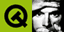DOC HOME SITE MAP MAN PAGES GNU INFO SEARCH PRINT BOOK
 Home | All Classes | Main Classes | Annotated | Grouped Classes | Functions# Format of the QDataStream Operators

The QDataStream allows you to serialize some of the Qt data types. The table below lists the data types that QDataStream can serialize and how they are represented.

It is best to always cast integers to a Qt integer type when writing, and to read back into the same Qt integer type when reading.

 Q_INT8 signed byte Q_INT16 signed 16 bit integer Q_INT32 signed 32 bit integer Q_UINT8 unsigned byte Q_UINT16 unsigned 16 bit integer Q_UINT32 unsigned 32 bit integer float 32-bit floating point number using the standard IEEE-754 format double 64-bit floating point number using the standard IEEE-754 format char * The size of the string including the terminating 0 (Q_UINT32) The string bytes including the terminating 0 The null string is represented as (Q_UINT32) 0. QBitArray The array size (Q_UINT32) The array bits, i.e. (size + 7)/8 bytes QBrush The brush style (Q_UINT8) The brush color (QColor) If style is CustomPattern, the brush pixmap (QPixmap) QByteArray The array size (Q_UINT32) The array bytes, i.e. size bytes QCString The size of the string including the terminating 0 (Q_UINT32) The string bytes including the terminating 0 The null string is represented as (Q_UINT32) 0. QColor RGB value serialized as a Q_UINT32 QColorGroup foreground (QBrush) button (QBrush) light (QBrush) midLight (QBrush) dark (QBrush) mid (QBrush) text (QBrush) brightText (QBrush) ButtonText (QBrush) base (QBrush) background (QBrush) shadow (QBrush) highlight (QBrush) highlightedText (QBrush) QCursor Shape id (Q_INT16) If shape is BitmapCursor: The bitmap (QPixmap), mask (QPixmap) and hot spot (QPoint) QDate Julian day (Q_UINT32) QDateTime Date (QDate) Time (QTime) QFont The family (QCString) The point size (Q_INT16) The style hint (Q_UINT8) The char set (Q_UINT8) The weight (Q_UINT8) The font bits (Q_UINT8) QImage If the image is null a "null image" marker is saved; otherwise the image is saved in PNG or BMP format (depending on the stream version). If you want control of the format, stream the image into a QBuffer (using QImageIO) and stream that. QMap The number of items (Q_UINT32) For all items, the key and value QPalette active (QColorGroup) disabled (QColorGroup) inactive (QColorGroup) QPen The pen styles (Q_UINT8) The pen width (Q_UINT8) The pen color (QColor) QPicture The size of the picture data (Q_UINT32) The raw bytes of picture data (char) QPixmap Save it as a PNG image. QPoint The x coordinate (Q_INT32) The y coordinate (Q_INT32) QPointArray The array size (Q_UINT32) The array points (QPoint) QRect left (Q_INT32) top (Q_INT32) right (Q_INT32) bottom (Q_INT32) QRegion The size of the data, i.e. 8 + 16 * (number of rectangles) (Q_UINT32) QRGN_RECTS (Q_INT32) The number of rectangles (Q_UINT32) The rectangles in sequential order (QRect) QSize width (Q_INT32) height (Q_INT32) QString If the string is null: 0xffffffff (Q_UINT32) otherwise: The string length (Q_UINT32) followed by the data in UTF-16 QTime Milliseconds since midnight (Q_UINT32) QValueList The number of list elements (Q_UINT32) All the elements in sequential order QVariant The type of the data (Q_UINT32) The data of the specified type QWMatrix m11 (double) m12 (double) m21 (double) m22 (double) dx (double) dy (double)